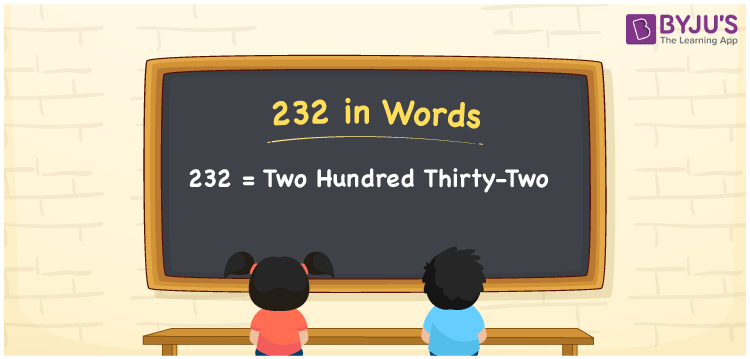# 232 in Words

We can write 232 in words as Two hundred thirty-two. If you bought a lotion that costs Rs. 232, then you can say, “I bought a lotion at Two hundred thirty-two rupees”. Thus, Two hundred thirty-two is called the number name or word form of 232. In this article, you will understand how to convert the cardinal number 232 into words with the help of a place value chart.

 232 in words Two hundred thirty-two Two hundred thirty-two in Numbers 232

## 232 in English Words

We generally express numbers in words using the English alphabet. So, we can spell 232 in English words as “Two hundred thirty-two”.## How to Write 232 in Words?

Let’s learn how to write the number 232 in words using a place value chart given here.

 Hundreds Tens Ones 2 3 2

Here, ones = 2, tens = 3, hundreds = 2

By expanding these numbers, we get;

2 × Hundred + 3 × Ten + 2 × One

= 2 × 100 + 3 × 10 + 2 × 1

= 200 + 30 + 2

= Two hundred + Thirty + Two

= Two hundred thirty-two

Therefore, 232 in words = Two hundred thirty-two

232 is a natural number that precedes 233 and succeeds 231.

232 in words – Two hundred thirty-two

Is 232 an odd number? – No

Is 232 an even number? – Yes

Is 232 a prime number? – No

Is 232 a composite number? – Yes

Is 232 a perfect square number? – No

Is 232 a perfect cube number? – No

## Frequently Asked Questions on 232 in Words

Q1

### How do you write 232 in words?

We can write the number 232 in words as Two hundred thirty-two.
Q2

### How to write Rs. 232 in words on a cheque?

On a cheque, Rs. 232 can be expressed in words as “Two hundred thirty-two rupees only”.
Q3

### Express the value of 232 + 1000 in word form.

232 + 1000 = 1232 Thus, we can express the value of 232 + 1000, i.e., 1232 in word form as One thousand two hundred thirty-two.